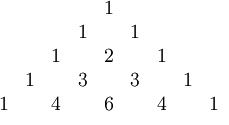HOME MATH DICTIONARY DOWNLOAD FEEDBACK DISCLAIMER
 Question: What is Pascal's Triangle ? Answer: Pascal's triangle is a geometric arrangement of the binomial coefficients in a triangle. Pascal’s triangle is a number pattern which starts with a 1 at the vertex, has a 1 at the end of each line, and each other number is found as the sum of the two numbers immediately above it. This triangular pattern is important in algebra and probability. The rows of Pascal's triangle are conventionally enumerated starting with row zero, and the numbers in odd rows are usually staggered relative to the numbers in even rows. A simple construction of the triangle proceeds in the following manner. On the zeroth row, write only the number 1. Then, to construct the elements of following rows, add the number directly above and to the left with the number directly above and to the right to find the new value. If either the number to the right or left is not present, substitute a zero in its place. For example, the first number in the first row is 0 + 1 = 1, whereas the numbers 1 and 3 in the third row are added to produce the number 4 in the fourth row. Pascal's triangle has higher dimensional generalizations. The three-dimensional version is called Pascal's pyramid or Pascal's tetrahedron, while the general versions are called Pascal's simplices.